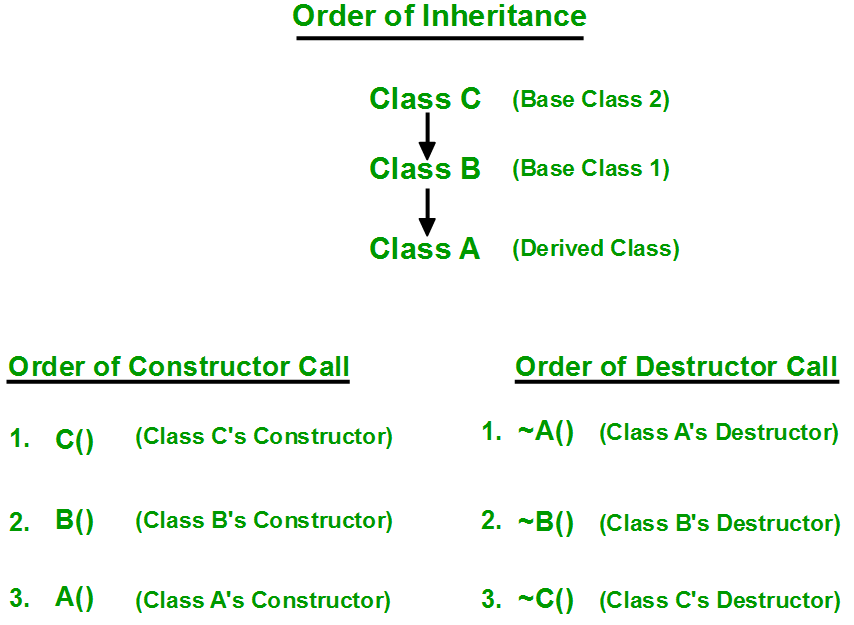# Order of Constructor/ Destructor Call in C++

Prerequisite: Constructors
Whenever we create an object of a class, the default constructor of that class is invoked automatically to initialize the members of the class.

If we inherit a class from another class and create an object of the derived class, it is clear that the default constructor of the derived class will be invoked but before that the default constructor of all of the base classes will be invoke, i.e the order of invocation is that the base class’s default constructor will be invoked first and then the derived class’s default constructor will be invoked.

Why the base class’s constructor is called on creating an object of derived class?

To understand this you will have to recall your knowledge on inheritance. What happens when a class is inherited from other? The data members and member functions of base class comes automatically in derived class based on the access specifier but the definition of these members exists in base class only. So when we create an object of derived class, all of the members of derived class must be initialized but the inherited members in derived class can only be initialized by the base class’s constructor as the definition of these members exists in base class only. This is why the constructor of base class is called first to initialize all the inherited members.

## C++

 `// C++ program to show the order of constructor call ` `// in single inheritance ` `  `  `#include ` `using` `namespace` `std; ` ` `  `// base class ` `class` `Parent ` `{ ` `    ``public``: ` `     `  `    ``// base class constructor ` `    ``Parent() ` `    ``{ ` `        ``cout << ``"Inside base class"` `<< endl; ` `    ``} ` `}; ` ` `  `// sub class ` `class` `Child : ``public` `Parent ` `{ ` `    ``public``: ` `     `  `    ``//sub class constructor ` `    ``Child() ` `    ``{ ` `        ``cout << ``"Inside sub class"` `<< endl; ` `    ``} ` `}; ` ` `  `// main function ` `int` `main() { ` `      `  `    ``// creating object of sub class ` `    ``Child obj; ` `     `  `    ``return` `0; ` `}  `

Output:

```Inside base class
Inside sub class```

Order of constructor call for Multiple Inheritance

For multiple inheritance order of constructor call is, the base class’s constructors are called in the order of inheritance and then the derived class’s constructor.

## C++

 `// C++ program to show the order of constructor calls  ` `// in Multiple Inheritance ` ` `  `#include ` `using` `namespace` `std; ` ` `  `// first base class ` `class` `Parent1 ` `{    ` `    `  `    ``public``: ` `     `  `    ``// first base class's Constructor     ` `    ``Parent1() ` `    ``{ ` `        ``cout << ``"Inside first base class"` `<< endl; ` `    ``} ` `}; ` ` `  `// second base class ` `class` `Parent2 ` `{ ` `    ``public``: ` `     `  `    ``// second base class's Constructor ` `    ``Parent2() ` `    ``{ ` `        ``cout << ``"Inside second base class"` `<< endl; ` `    ``} ` `}; ` ` `  `// child class inherits Parent1 and Parent2 ` `class` `Child : ``public` `Parent1, ``public` `Parent2 ` `{ ` `    ``public``: ` `     `  `    ``// child class's Constructor ` `    ``Child() ` `    ``{ ` `        ``cout << ``"Inside child class"` `<< endl; ` `    ``} ` `}; ` ` `  `// main function ` `int` `main() { ` `     `  `    ``// creating object of class Child ` `    ``Child obj1; ` `    ``return` `0; ` `}  `

Output:

```Inside first base class
Inside second base class
Inside child class```

Order of constructor and Destructor call for a given order of InheritanceHow to call the parameterized constructor of base class in derived class constructor?

To call the parameterized constructor of base class when derived class’s parameterized constructor is called, you have to explicitly specify the base class’s parameterized constructor in derived class as shown in below program:

## C++

 `// C++ program to show how to call parameterized Constructor ` `// of base class when derived class's Constructor is called ` ` `  `#include ` `using` `namespace` `std; ` ` `  `// base class ` `class` `Parent { ` `    ``int` `x; ` ` `  `public``: ` `    ``// base class's parameterized constructor ` `    ``Parent(``int` `i) ` `    ``{ ` `        ``x = i; ` `        ``cout << ``"Inside base class's parameterized "` `                ``"constructor"` `             ``<< endl; ` `    ``} ` `}; ` ` `  `// sub class ` `class` `Child : ``public` `Parent { ` `public``: ` `    ``// sub class's parameterized constructor ` `    ``Child(``int` `x): Parent(x) ` `    ``{ ` `        ``cout << ``"Inside sub class's parameterized "` `                ``"constructor"` `             ``<< endl; ` `    ``} ` `}; ` ` `  `// main function ` `int` `main() ` `{ ` ` `  `    ``// creating object of class Child ` `    ``Child obj1(10); ` `    ``return` `0; ` `}`

Output:

```Inside base class's parameterized constructor
Inside sub class's parameterized constructor```

Important Points

• Whenever the derived class’s default constructor is called, the base class’s default constructor is called automatically.
• To call the parameterized constructor of base class inside the parameterized constructor of sub class, we have to mention it explicitly.
• The parameterized constructor of base class cannot be called in default constructor of sub class, it should be called in the parameterized constructor of sub class.

Destructors in C++ are called in the opposite order of that of Constructors.
If you like GeeksforGeeks and would like to contribute, you can also write an article using write.geeksforgeeks.org or mail your article to review-team@geeksforgeeks.org. See your article appearing on the GeeksforGeeks main page and help other Geeks.